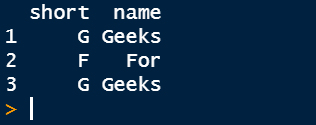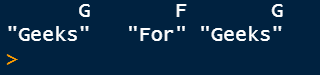# Convert two columns of a data frame to a named vector in R

• Last Updated : 01 Apr, 2021

In this article, we will discuss how to convert two columns of a dataframe to a named vector in R Programming Language.

Let see the implementation stepwise:

Example 1: Creating dataframe and convert columns to vector.

Step 1: Here we create a DataFrame with name data. There is a total of two columns in DataFrame, short and name. To create a data frame, we use data.frame() function and then finally checkout our DataFrame by printing it.

Code:

## R

 `short = ``c``(``"G"``,``"F"``,``"G"``)`` ` `name = ``c``(``"Geeks"``, ``"For"``, ``"Geeks"``)`` ` `data = ``data.frame``(short, name)``print``(data)`

Output:Step 2: Convert dataframe columns to a vector called result by use of setNames() function. Inside setNames(), we use as.character() function. For access vectors from DataFrame, we use \$ operator and then finally print it.

• setNames(object): it is a function in R that sets a name on an object and returns the object.
• as.character(vector): it is the function that returns a string of a character vector and prints out the string representation.

Code:

## R

 `short = ``c``(``"G"``,``"F"``,``"G"``)``name = ``c``(``"Geeks"``, ``"For"``, ``"Geeks"``)``data = ``data.frame``(short, name)`` ` `result <- ``setNames``(``as.character``(data\$name), ``                   ``as.character``(data\$short))``print``(result)`

Output:Example 2: Now, we take another example for better understanding. Here we use srno and student_name as two columns of student data DataFrame and get the final result in the approach vector.

## R

 `srno = ``c``(1:5)``student_name = ``c``(``"John"``, ``"Jane"``, ``"Bill"``, ``"Jeff"``, ``"Elon"``)``studentdata = ``data.frame``(srno, student_name)``#print(studentdata)`` ` `approach <- ``setNames``(``as.character``(studentdata\$student_name),``                     ``studentdata\$srno)``print``(approach)`

Output:My Personal Notes arrow_drop_up